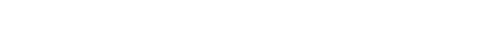glFog.3glOpenGL man pages

```

```

## Name

```  glFogf, glFogi, glFogfv, glFogiv - specify fog parameters

```

## C Specification

```  void glFogf( GLenum pname,
GLfloat param )
void glFogi( GLenum pname,
GLint param )

```

## Parameters

```
pname	  Specifies a single-valued fog	parameter.  GL_FOG_MODE,
GL_FOG_DENSITY, GL_FOG_START,	GL_FOG_END, and	GL_FOG_INDEX are
accepted.

param	  Specifies the	value that pname will be set to.

```

## C Specification

```  void glFogfv(	GLenum pname,
const GLfloat *params )
void glFogiv(	GLenum pname,
const GLint *params )

```

## Parameters

```
pname
Specifies a fog parameter.  GL_FOG_MODE,	GL_FOG_DENSITY,	GL_FOG_START,
GL_FOG_END, GL_FOG_INDEX, and GL_FOG_COLOR are accepted.

params
Specifies the value or values to	be assigned to pname.  GL_FOG_COLOR
requires	an array of four values.  All other parameters accept an
array containing	only a single value.

```

## Description

```  Fog is enabled and disabled with glEnable and	glDisable using	the argument
GL_FOG.  While enabled, fog affects rasterized geometry, bitmaps, and	pixel
blocks, but not buffer clear operations.

glFog	assigns	the value or values in params to the fog parameter specified
by pname.  The accepted values for pname are as follows:

GL_FOG_MODE	      params is	a single integer or floating-point value that
specifies	the equation to	be used	to compute the fog
blend factor, f.	Three symbolic constants are
accepted:	GL_LINEAR, GL_EXP, and GL_EXP2.	 The
equations	corresponding to these symbolic	constants are
defined below.  The default fog mode is GL_EXP.

GL_FOG_DENSITY      params is	a single integer or floating-point value that
specifies	density, the fog density used in both
exponential fog equations.  Only nonnegative densities
are accepted.  The default fog density is	1.0.

GL_FOG_START	      params is	a single integer or floating-point value that
specifies	start, the near	distance used in the linear
fog equation.  The default near distance is 0.0.

GL_FOG_END	      params is	a single integer or floating-point value that
specifies	end, the far distance used in the linear fog
equation.	 The default far distance is 1.0.

GL_FOG_INDEX	      params is	a single integer or floating-point value that
specifies	i , the	fog color index.  The default fog
f
index is 0.0.

GL_FOG_COLOR	      params contains four integer or floating-point values
that specify C , the fog color.  Integer values are
f
mapped linearly such that	the most positive
representable value maps to 1.0, and the most negative
representable value maps to -1.0.	 Floating-point
values are mapped	directly.  After conversion, all
color components are clamped to the range	[0,1].	The
default fog color	is (0,0,0,0).

Fog blends a fog color with each rasterized pixel fragment's posttexturing
color	using a	blending factor	f.  Factor f is	computed in one	of three
ways,	depending on the fog mode.  Let	z be the distance in eye coordinates
from the origin to the fragment being	fogged.	 The equation for GL_LINEAR
fog is

end - z
f = -----------
end	- start

The equation for GL_EXP fog is

-(density · z)
f = e

The equation for GL_EXP2 fog is

2
-(density · z)
f = e

Regardless of	the fog	mode, f	is clamped to the range	[0,1] after it is
computed.  Then, if the GL is	in RGBA	color mode, the	fragment's color Cr
is replaced by

C' = fC +(1-f)C
r     r       f

In color index mode, the fragment's color index i  is	replaced by
r

i' = i +(1-f)i
r    r       f
```

## Errors

```  GL_INVALID_ENUM is generated if pname	is not an accepted value, or if	pname
is GL_FOG_MODE and params is not an accepted value.

GL_INVALID_VALUE is generated	if pname is GL_FOG_DENSITY and params is
negative.

GL_INVALID_OPERATION is generated if glFog is	called between a call to
glBegin and the corresponding	call to	glEnd.

```

## Associated Gets

```  glIsEnabled with argument GL_FOG
glGet	with argument GL_FOG_COLOR
glGet	with argument GL_FOG_INDEX
glGet	with argument GL_FOG_DENSITY
glGet	with argument GL_FOG_START
glGet	with argument GL_FOG_END
glGet	with argument GL_FOG_MODE

```

```  glEnable